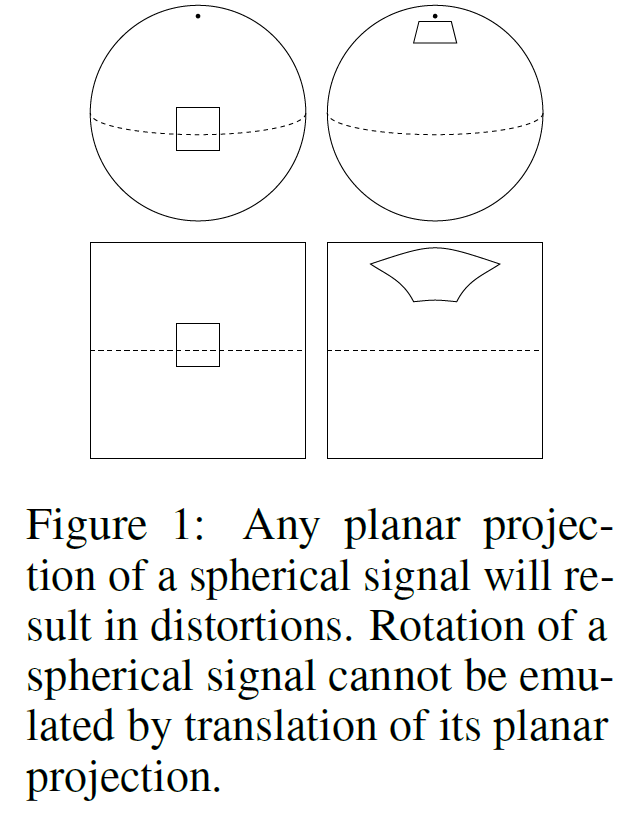# 三维卷积：全景图像Spherical CNNs（Code）

卷积神经网络（CNN）可以很好的处理二维平面图像的问题。然而，对球面图像进行处理需求日益增加。例如，对无人机、机器人、自动驾驶汽车、分子回归问题、全球天气和气候模型的全方位视觉处理问题。

将球形信号的平面投影作为卷积神经网络的输入的这种Too Naive做法是注定要失败的，Cnns的巨大成就来源于局部感受野的权值共享，而多层结构总能找到不同rect的相同目标，给出响应。而对于球形图像，一个目标在图片的不同位置是发生形变的，若要使用CNNs直接共享，构建的局部感受野理应描述这种转换。如下图所示，而这种平面投影引起的空间扭曲会导致CNN无法共享权重。We propose a definition for the spherical cross-correlation that is both expressive and rotation-equivariant. The spherical correlation satisfies a generalized Fourier theorem, which allows us to compute it efficiently using a generalized(non-commutative) Fast Fourier Transform (FFT) algorithm. We demonstrate the computational efficiency, numerical accuracy, and effectiveness of spherical CNNs applied to 3D model recognition and atomization energy regression.

? 如何使三维图像由二维图像重构出来，解决在不同位置产生形变问题，经典的FFT方法和李群模型就成为这种桥梁。

? 关于SO3 作为刚体变换的阐述，参考：半闲居士视觉SLAM十四讲笔记(3)三维空间刚体运动 - par..._CSDN博客 。

? wocao，这个大纲写的更简洁明了：高翔《视觉SLAM十四讲》从理论到实践。

? ? 区分出三维图像和平面的细微差别，把球面图像看做是三维流形，把球面展开为离散的三维李群，把SO(3)的关系用CNNs的高层进行表示。

? ? As shown in Figure 1, there is no good way to use translational convolution or cross-correlation1 to analyze spherical signals. The most obvious approach, then, is to change the definition of crosscorrelation by replacing filter translations by rotations. Doing so, we run into a subtle but important difference between the plane and the sphere: whereas the space of moves for the plane (2D translations) is itself isomorphic to the plane, the space of moves? for the sphere (3D rotations) is a different, three-dimensional manifold called SO(3)2. It follows that the result of a spherical correlation (the output feature map) is to be considered a signal on SO(3), not a signal on the sphere, S2. For this reason, we deploy SO(3) group correlation in the higher layers of a spherical CNN (Cohen and Welling, 2016).

? ? The implementation of a spherical CNN (S2-CNN) involves two major challenges. Whereas a square grid of pixels has discrete translation symmetries, no perfectly symmetrical grids for the sphere exist. This means that there is no simple way to define the rotation of a spherical filter by one pixel. Instead, in order to rotate a filter we would need to perform some kind of interpolation. The other challenge is computational efficiency; SO(3) is a three-dimensional manifold, so a naive implementation of SO(3) correlation is O(n6).